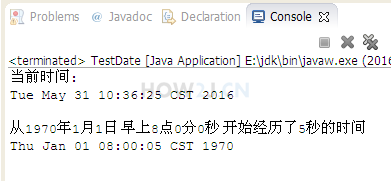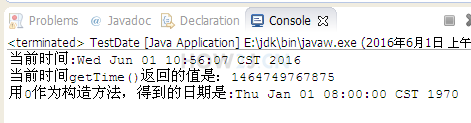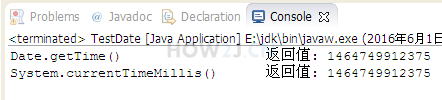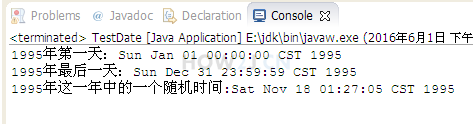Date类9分38秒

## 创建日期对象package date; // import java.util.Date; public class TestDate { public static void main(String[] args) { // 当前时间 Date d1 = new Date(); System.out.println("当前时间:"); System.out.println(d1); System.out.println(); // 从1970年1月1日 早上8点0分0秒 开始经历的毫秒数 Date d2 = new Date(5000); System.out.println("从1970年1月1日 早上8点0分0秒 开始经历了5秒的时间"); System.out.println(d2); } }

## getTime

getTime() 得到一个long型的整数package date; // import java.util.Date; public class TestDate { public static void main(String[] args) { //注意：是java.util.Date; //而非 java.sql.Date，此类是给数据库访问的时候使用的 Date now= new Date(); //打印当前时间 System.out.println("当前时间:"+now.toString()); //getTime() 得到一个long型的整数 //这个整数代表 1970.1.1 08:00:00:000，每经历一毫秒，增加1 System.out.println("当前时间getTime()返回的值是："+now.getTime()); Date zero = new Date(0); System.out.println("用0作为构造方法，得到的日期是:"+zero); } }

## System.currentTimeMillis()

new Date().getTime() 和 System.currentTimeMillis() 是一样的package date; // import java.util.Date; public class TestDate { public static void main(String[] args) { Date now= new Date(); //当前日期的毫秒数 System.out.println("Date.getTime() \t\t\t返回值: "+now.getTime()); //通过System.currentTimeMillis()获取当前日期的毫秒数 System.out.println("System.currentTimeMillis() \t返回值: "+System.currentTimeMillis()); } }
```package date;

//
import java.util.Date;

public class TestDate {

public static void main(String[] args) {
Date now= new Date();

//当前日期的毫秒数
System.out.println("Date.getTime() \t\t\t返回值: "+now.getTime());
//通过System.currentTimeMillis()获取当前日期的毫秒数
System.out.println("System.currentTimeMillis() \t返回值: "+System.currentTimeMillis());

}
}
```

## 练习-日期

姿势不对,事倍功半! 点击查看做练习的正确姿势## 答案-日期6分52秒 本视频采用html5方式播放，如无法正常播放，请将浏览器升级至最新版本，推荐火狐，chrome，360浏览器。 如果装有迅雷，播放视频呈现直接下载状态，请调整 迅雷系统设置-基本设置-启动-监视全部浏览器 (去掉这个选项)。 chrome 的 视频下载插件会影响播放，如 IDM 等，请关闭或者切换其他浏览器

package date; import java.util.Date; public class TestDate { public static void main(String[] args) { long second = 1000; long minute = 60*second; long hour = minute * 60; long day = hour*24; long year = day * 365; long year1995Start = (1995-1970) * year; long leapDay = (1995-1970)/4*day; //每隔4年有一个润日 year1995Start+=leapDay; long eightHour = hour*8; //8个小时的毫秒数，因为从0毫秒对应的是1970.1.1 08:00:00 year1995Start-=eightHour; Date dStart = new Date(year1995Start); System.out.println("1995年第一天："+dStart); long year1995End = year1995Start + year - 1; Date dEnd = new Date(year1995End); System.out.println("1995年最后一天："+dEnd); long randomTime = (long) (Math.random()*(year-1) + year1995Start); Date dRandom = new Date(randomTime); System.out.println("1995年这一年中的一个随机时间:" + dRandom); } }

HOW2J公众号，关注后实时获知最新的教程和优惠活动，谢谢。2022-07-07 简便答案
 tumaowolf 关于 JAVA 基础-日期-Date 的提问 答案 ```public static void main(String[] args) throws ParseException { SimpleDateFormat format = new SimpleDateFormat("yy-MM-dd HH:mm:ss"); String start = "1995-01-01 00:00:00"; String end = "1995-12-31 23:59:59"; Long ceil = format.parse(start).getTime(); Long floor = format.parse(end).getTime(); Long number = (long)(Math.random()*(floor-ceil)) + ceil; System.out.println(new Date(number)); }```

2 个答案

ljc20020822

package date; import java.text.SimpleDateFormat; import java.util.Calendar; import java.util.Date; public class TestDate1 { public static void main(String[] args) { //y 代表年 //M 代表月 //d 代表日 //h 代表24进制的小时 //m 代表分钟 //s 代表秒 //S 代表毫秒 SimpleDateFormat sdf = new SimpleDateFormat("yyyy-MM-dd HH:mm:ss SSS"); Date min = new Date(70,1,1,8,0,0); long mindate = min.getTime(); Date max = new Date(95,12,31,23,59,59); long maxdate = max.getTime(); long radndom =(long)((Math.random())*(maxdate-mindate)+mindate); Date randomdate = new Date(radndom); String str=sdf.format(randomdate); System.out.println(str); } }

keiven

package date; import java.util.Date; public class TestDate03 { public static void main(String[] args) { Date d =new Date(); System.out.println(d.getTime()); Date min =new Date(70,1,1,8,0,0); long mindate =min.getTime(); Date max=new Date(95,12,31,23,59,59); long maxdate =max.getTime(); System.out.println(mindate); long random=(long)((Math.random())*(maxdate-mindate)+mindate); Date randomdate =new Date(random); System.out.println(randomdate); } }

2022-05-11 把功能封装起来了，写的内容很多
 芬达味橘猫 关于 JAVA 基础-日期-Date 的提问 先设定一个开始时间start_timeStamp和一个结束时间戳end_timeStamp 随机数取这个之间的范围，最终通过时间戳转换日期，完成随机生成这个日期 ```import java.util.Date; import java.text.ParseException; import java.text.SimpleDateFormat; public class randomTime{ /** 先设定一个开始时间start_timeStamp和一个结束时间戳end_timeStamp 随机数取这个之间的范围，最终通过时间戳转换日期，完成随机生成这个日期 */ private String timeStr = ""; private static String time_pattern = "yyyy-MM-dd HH:mm:ss"; SimpleDateFormat sdf = new SimpleDateFormat(time_pattern); private Date parse_time = null; public randomTime(){ } public randomTime(String dateStr){ this.timeStr = dateStr; } //解析时用字符串转时间 public Date createRandomTime(){ try { Date parse_time = sdf.parse(timeStr); this.parse_time = parse_time; return parse_time; } catch (ParseException e) { e.printStackTrace(); } return null; } public Date timestampToDate(long timeStamp){ String date_string = sdf.format(timeStamp); try { Date toDate = sdf.parse(date_string); return toDate; } catch (ParseException e) { e.printStackTrace(); } return null; } //转成时间戳 public long getTimeStamp(){ long timeStamp= parse_time.getTime(); return timeStamp; } public static void main(String[] args) { randomTime start = new randomTime("1995-01-01 00:00:00"); //输出时间 System.out.println("1995年的第一天为："+start.createRandomTime()); long start_timeStamp= start.getTimeStamp(); //定义1995最后一天 randomTime end = new randomTime("1995-12-31 23:59:59"); System.out.println("1995年的最后一天为："+end.createRandomTime()); long end_timeStamp= end.getTimeStamp(); //生成一个时间戳随机数（0.0-0.99）* （最大时间-最小时间的区间）+（最小时间） long random_timeStamp = (long) (Math.random()*(end_timeStamp - start_timeStamp+1)+(start_timeStamp)); randomTime random_time = new randomTime(); Date rtd = random_time.timestampToDate(random_timeStamp); System.out.println("1995年这一年中的一个随机时间："+rtd); } }```

1 个答案

keiven

package date; import java.util.Date; import java.util.Arrays; public class TestDate05 { public static void main(String[] args) { long [] a=new long; Date d1 = new Date(0); long mindate=d1.getTime(); Date d2=new Date(100,1,1); long maxdate=d2.getTime(); System.out.println(mindate); System.out.println(maxdate); for(int i=0;i<=8;i++) { long t=0; t = (long) (Math.random() * (maxdate - mindate) + mindate); a[i]=t; } Arrays.sort(a); for(int i=0;i<=8;i++) { Date date = new Date(a[i]); System.out.println("排序的日期为："+date.toString()); } } }

2022-05-11 答案
2022-02-25 答案
2022-01-09 答案